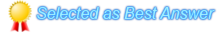You must Sign In to post a response.
• # How to Round of Input values Text Box using C#

Hi Developers;

When i am try to Round of the TextBox values i got error.

Following is my code

double a = txt1.Text; ==> Cannot Implicitly Convert type 'string' to 'double' =(Error)
string[] str1 = a.ToString().Split('.');
double num1 = Convert.ToDouble("0." + str1);
double res;
if (num1 < 0.51)
{
res = Math.Floor(a);
}
else
{
res = Math.Round(a);
}

So how i am resolve this error.
How to save the input textbox value with Roundof.
The code will be in C# as Well.

Thanks with

Paul.S
•Do not try to convert string value to double directly, use Convert.ToDouble Method, it will Converts the specified string representation of a number to an equivalent double-precision floating-point number.

Try
{
double result = Convert.ToDouble(txt1.Text);
}
Catch (Exception ex)
{
//value is not a number
}

Thanks
Koolprasd2003
Editor, DotNetSpider MVM
Microsoft MVP 2014 [ASP.NET/IIS]

• just change
double a = txt1.Text; ==> Cannot Implicitly Convert type 'string' to 'double' =(Error)

to

string a = txt1.Text;

then it should work without compile errors.

Thanks,
Murali.

Regards,
Muralidhar.

•your code after is as follows
string a = txt1.Text; // ==> Cannot Implicitly Convert type 'string' to 'double' =(Error)
string[] str1 = a.Split('.');

double num1 = Convert.ToDouble(str1);
double res;
if (num1 < 0.51)
{
res = Math.Floor(Convert.ToDouble(a));
}
else
{
res = Math.Round(Convert.ToDouble(a));
}
Console.WriteLine(a.ToString());

Hope this helps.

Thanks,
Murali.

Regards,
Muralidhar.

• Hai Paul,
As the other answers are mentioned, the error "double a = txt1.Text; ==> Cannot Implicitly Convert type 'string' to 'double' =(Error)" is due to that you are assigning the string value to the double so it is throwing error.
You need to assign it to string and then do all your stuff.
Hope it will be helpful to you.

Regards,
Pawan Awasthi(DNS MVM)
+91 8123489140 (whatsApp), +60 14365 1476(Malaysia)
pawansoftit@gmail.com

• Hi Paul,

In your code the below piece is wrong.

`double a = txt1.Text;`

While compare 2 parameters, both should be in same format, but in your code left side value is in double format and right side value is in string format, so it gives error while comparing like " Cannot Implicitly Convert type 'string' to 'double'",

to overcome the above error, you need to convert any one side value to some other,

I just convert your right side value into double format.

`double a = Convert.ToDouble( txt1.Text);`
Now, this will give you the result what you are expected.

Hope this will helpful to you....

--------------------------------------------------------------------------------
Give respect to your work, Instead of trying to impress your boss.

N@veen
Blog : http://naveens-dotnet.blogspot.in/

• Thanks a lot Mr.Naveen

Paul.S

• Your code should be:
String a = txt1.Text;
string[] str1 = a.ToString().Split('.');
double num1 = Convert.ToDouble("0." + str1);
double res;
if (num1 < 0.51)
{
res = Math.Floor(a);
}
else
{
res = Math.Round(a);
}

If you are unable to do something properly for the first time, call it Version 1.0!

Thanks & Regards,
MONOJ BHUINA

• Tahnks a Lot Mr.Muralidhar. God bless you

Paul.S